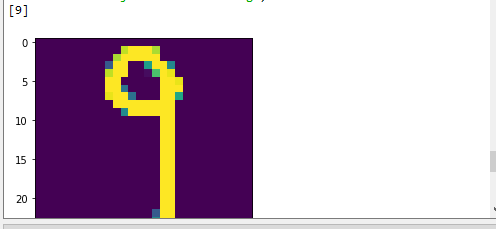# Your first step in deep learning

Source: Deep Learning on Medium# Handwritten Digit Recognition using Convolutional Neural Networks in Python with Keras

The first step of object recognition for machine learning and deep learning is the MNIST dataset for handwritten digit recognition.

In this articl you will discover how to develop a deep learning model to achieve near state of the art performance on the MNIST handwritten digit recognition task in Python using the Keras deep learning library.

# After completing this tutorial, you will know:

1- How to load the MNIST dataset in Keras.

2- How to develop and evaluate a baseline neural network model for the MNIST problem.

3- How to implement and evaluate a simple Convolutional Neural Network for MNIST.

4- discover the different components of CNN with their implementation in python with keras model.

# context :

In this article, we will show how to predict an image on a trained keras model. Our objective was therefore to build a CNN which can identify the number shown in our image (we will build our test image with paint, we will draw the number manually) and save the model as an HDF5 file. So later on, you may want to take this model and give it your own handwritten numbers images and you want to see if it is able to accurately identify these images.

# Let’s start in our project :

## DATASET used for learning:

Base de données MNIST de chiffres manuscrits
Ensemble de données de 60 000 images en niveaux de gris 28 x 28 des 10 chiffres, avec un ensemble de test de 10 000 images.
Use:

`from keras.datasets import mnist(x_train, y_train), (x_test, y_test) = mnist.load_data()`

Back :

2 tuples:
x_train, x_test: uint8 grayscale image data table with shape (num_samples, 28, 28).
– y_train, y_test: uint8 array of number labels (integers between 0 and 9) with a form (num_samples,).

Arguments:

chemin : si vous n’avez pas le fichier d’index localement (at `'~/.keras/datasets/' + path`), il sera téléchargé à cet emplacement.

## The first step is to import the necessary classes and functions:

In Keras, the layers used for two-dimensional convolutions expect pixel values with the dimensions [pixels] [width] [height] [channels].
In the case of RGB, the last pixel dimension would be 3 for the red, green and blue components and it would be like having 3 image inputs for each color image. In the case of MNIST where the pixel values are gray levels, the pixel size is set to 1.

As before, it is a good idea to normalize the pixel values in the range 0 and 1 and hot coding the output variables :

We define a large CNN architecture with additional convolutional grouping layers and fully connected layers. The network topology can be summarized as follows:
1- The first hidden layer is a convolutional layer called Convolution2D. The layer has 32 feature cards, which have a size of 5 × 5 and a rectifier activation function. This is the input layer, which expects images with the outline of the structure above [pixels] [width] [height].
2- Next, we define a grouping layer which takes the max called MaxPooling2D. It is configured with a pool size of 2 × 2.
3- Convolutional layer with 15 characteristic cards of size 3 × 3.
4- Pooling layer taking at most more than 2 * 2 patches.
5- The next layer is a regularization layer using the dropout called Dropout. It is configured to randomly exclude 20% of neurons from the layer in order to reduce over-adjustment.
6- Next comes a layer which converts the data from the 2D matrix into a vector called Flatten. It allows the output to be processed by fully connected standard layers.
7- Fully connected layer with 128 neurons and activation of the rectifier.
8- Fully connected layer with 50 neurons and activation of the rectifier.
9- Output layer.

## for the training phase :

The model is suitable for 45 eras with a lot size of 200.

## Then save the result of our model in a “.h5” file :

Keras provides a basic backup format using the HDF5 standard. The saved model can be treated as a single binary blob.
Keras allows you to export a model and an optimizer to a file so that it can be used without access to the original python code. It contains weights, variables and the configuration of the model. Since the optimizer state is recovered, you can even resume training exactly where you left off.
You can use model.save (filepath) to save a Keras model in a single HDF5 file which will contain:
• the weights of the model.
• training configuration (loss, optimizer)
• optimizer status, allowing you to resume training exactly where you left off

Then we can save our model with the following line of code:

# And now our model is ready for use at the prediction :

When previewing a single image, you should reshape the image even if you only have one. Your entry must be in the form:

[1, image_width, image_height, number_of_channels]

Then we will create a new python file for the tests (to avoid repetition of the learning phase of our model) :

We load our model to save in “.h5” format:

We load our image and we prepare it for the prediction:

We predict and display the result:

# Now we will test our program:

We open paint we draw a number in white with a black backgroud with the dimensions mentioned before:

And finally we launch our prediction program:

# follow and contact me :

Instagram : https://bit.ly/2RqfZZ5